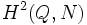# Supergroups of Klein four-group

## Contents

View supergroups of particular groups | View other specific information about Klein four-group

Note that unlike the discussion of the subgroup structure of Klein four-group, thisdiscussion is necessarily not comprehensive, because there are infinitely many groups containing the Klein four-group as a subgroup.

## General lists

### Direct products

Each of the groups listed below arises as the external direct product of Klein four-group and some nontrivial group. In particular, each of these groups contains the Klein four-group as a direct factor -- and hence as both a normal subgroup and a quotient group.

Note that since order of direct product is product of orders, if the other group has order$a$, the direct product has order$4a$.

Other factor of direct product Order Second part of GAP ID Value of direct product Order Second part of GAP ID Hall-Senior symbol (if applicable)
cyclic group:Z2 2 1 elementary abelian group:E8 8 5$(1)^3$
cyclic group:Z3 3 1 direct product of Z6 and Z2 12 5
cyclic group:Z4 4 1 direct product of Z4 and V4 16 10$1^22$
Klein four-group 4 2 elementary abelian group:E16 16 14$1^4$
cyclic group:Z5 5 1 direct product of Z10 and Z2 20 5
symmetric group:S3 6 1 direct product of D12 and Z2 24 14
cyclic group:Z6 6 2 direct product of E8 and Z3 24 15

## Classification of supergroups of order eight

### The general procedure

Suppose$G$ is a group of order eight containing a subgroup$N$ of order four isomorphic to the Klein four-group. Note that since$N$ is of index two, it is a normal subgroup. Further, the quotient group is isomorphic to$Q$, the cyclic group of order two.

The classification has two steps:

• Find all homomorphisms from$Q$ to$\operatorname{Aut}(N)$.
• For each such homomorphism, find all possible extensions. These are classified by the elements of$H^2(Q,N)$ for the action.

### The classification$\operatorname{Aut}(N)$ is isomorphic to the symmetric group of degree three. This has three subgroups of order two, all of which are conjugate. Thus, up to equivalence under automorphisms of$N$, there is only one subgroup of order two.

Thus, there are (up to equivalence under automorphisms) two homomorphisms from$Q$ to$\operatorname{Aut}(N)$: the trivial homomorphism and an isomorphism to one of the subgroups of order two.

For the trivial map, the cohomology group$H^2(Q,N)$ has order two, and the two extensions are:

• elementary abelian group of order eight: This corresponds to the identity element of$H^2(Q,N)$.
• direct product of Z4 and Z2: This corresponds to the non-identity element of$H^2(Q,N)$.

For the nontrivial map, the cohomology group$H^2(Q,N)$ is trivial, and the unique extension corresponding to it is the dihedral group of order eight.